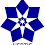## Saturday, January 31, 2009

### Elearn Geometry Problem 236: Quadrilateral, Midpoints, Exterior line, Perpendicular linesSee complete Problem 236 at:

Level: High School, SAT Prep, College geometry

1.macsim gabriel florin has left a new comment on your post "Elearn Geometry Problem 236: Quadrilateral, Midpoints...":

Let A' , B', C' and D' the projections of A, B, C, D on the exterior line.We obtain the trapeziods AA'B'B, BB'C'C, CC'D'D, DD'A'A. In this trpezoids we have :
e=(AA'+BB')/2
f=(BB'+CC')/2
g=(CC'+DD')/2
h=(DD'+AA')/2
From this relations results that e+g=f+h

2.See the drawing

- From problem 146, EH//FG => E’H’=F’G’
- Draw E’’H’//EH, E’’ on EE’ =>E’E’’=e-h
- Draw F’’G’//FG, F’’ on FF’ => F’F’’=f-g
- E’’H’//EH, F’’G’//FG and EH//FG => E’’H’//F’’G’
- =>E’’E’H’ is similar to F’’F’G’
- E’H’=F’G’ => E’’E’H’ is congruent to F’’F’G’
- =>E’E’’=F’F’’
-=>e-h=f-g
Therefore e+g=f+h